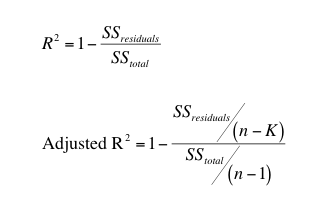# Statistics Resources

This guide contains all of the ASC's statistics resources. If you do not see a topic, suggest it through the suggestion box on the Statistics home page.

## Coefficient of Determination (R-square)

Coefficient of Determination

When looking at relationships through a correlation or regression analysis, we often use the coefficient of determination as a measure of effect size. This is also referred to as R-squared. In a multiple linear regression analysis, we typically use the adjusted R-squared value.

Computing R-Squared

As with Cohen's d, we can compute the R-squared value using a formula. There are several different formulas that can be used. Here is just one example:where:

• SS stands for "sum of squares"
• n represents the sample size
• K represents the number of parameters in the model

In order to complete this computation, you must also know how to compute each sum of squares (deviations from the mean), which can be a time-consuming process, especially with larger data sets. Thankfully, like with Cohen's d, we can also use technology to compute the R-Squared.

R-Squared using SPSS

1) Correlations

Computing R-Squared when conducting a correlation analysis is pretty simple. When you run a correlation analysis, you are computing "r", the correlation coefficient. In order to obtain R-Squared, we simply square the correlation value, that is we multiply the correlation coefficient by itself. See the correlation guide for help with running a correlation analysis.

2) Regression

Each time you conduct a regression analysis in SPSS, a table will automatically be included that contains the R-Square and Adjusted R-Square values, as shown below:When reporting the results for our analysis, we would include that the R2 = .305. This means that approximately 30.5% of the variability in the outcome variable is explained by the regression model.

Interpreting the Coefficient of Determination

The coefficient of determination tells us how much variability in the outcome/dependent variable is explained by the relationship between the variables. In a correlation, that relationship is only between the two variables. In a regression analysis, it's referring to the regression model as a whole.

You'll notice that when conducting a simple linear regression, with only 1 predictor and 1 outcome variable, the R-square value is the same as what you would get if you run a simple correlation analysis between those two variables. This is because the model is based on the relationship between only those two variables.

**For additional assistance with computing and interpreting the effect size for your analysis, attend the SPSS: Correlation/Linear Regression group session**